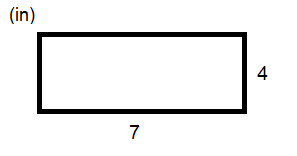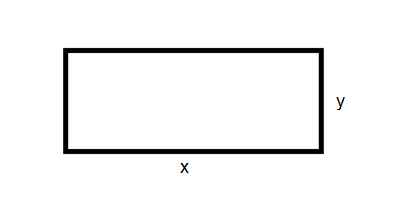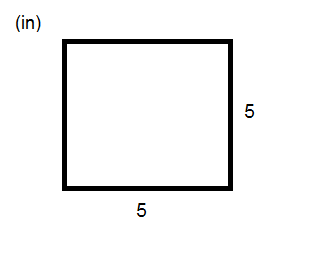# Area and Perimeter Formulas, Definition and Examples

0
357

## Calculating the Area and the Perimeter

P=x+x+y+y
P=2x+2y
P=2(x+y)

#### Find the perimeter of this rectangle:#### The area is measurement of the surface of a shape. To find the area of a rectangle or a square you need to multiply the length and the width of a rectangle or a square.#### Find the area of this square.#### There are different units for perimeter and area The perimeter has the same units as the length of the sides of rectangle or square whereas the area’s unit is squared.

READ HERE  Area Under the Curve Formula with Solved Example